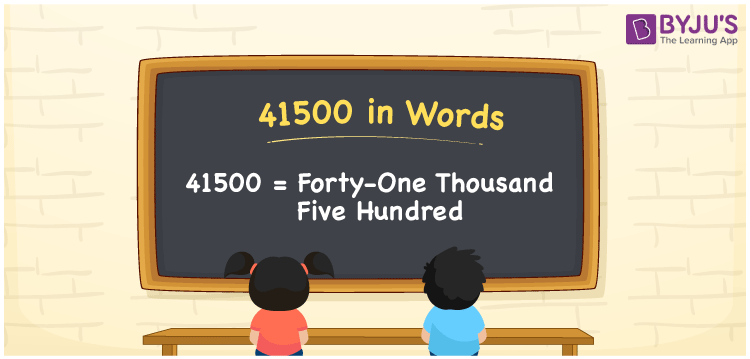# 41500 in Words

41500 in words is written as Thirteen thousand four hundred. In both the International System of Numerals and the Indian System of Numerals, 41500 in words is written as Forty-one thousand five hundred. The number 41500 is a Cardinal Number as it represents some quantity. For example, “this television costs 41500 rupees”.

 41500 in words Forty-one thousand five hundred Forty-one thousand five hundred in number 41500

## 41500 in English Words

41500 in English words is read as “Forty-one thousand five hundred.”## How to Write 41500 in Words?

To write 41500 in words, we shall use the place value chart. In the place value chart, put 4 in the ten thousands, 1 in the thousands, 5 in the hundreds, and 0 in the tens and the ones, respectively. Let us make a place value chart to write the number 41500 in words.

 Ten Thousands Thousands Hundreds Tens Ones 4 1 5 0 0

Thus, we can write the expanded form as

4 × Ten Thousand + 1 × Thousand + 5 × Hundred + 0 × Ten + 0 × One

= 4 × 10000 + 1 × 1000 + 5 × 100 + 0 × 10 + 0 × 1

= 40000 + 1000 + 500 + 0 + 0

= 41500

= Forty-one thousand five hundred.

41500 is a natural number between 41499 and 41501.

41500 in words – Forty-one thousand five hundred

• Is 41500 an odd number? – No
• Is 41500 an even number? – Yes
• Is 41500 a perfect square number? – No
• Is 41500 a perfect cube number? – No
• Is 41500 a prime number? – No
• Is 41500 a composite number? – Yes

## Frequently Asked Questions on 41500 in Words

Q1

### How to write 41500 in words?

41500 in words is written as Forty-one thousand five hundred.
Q2

### How to write 41500 in words in the International and Indian System of Numerals?

In both, the system of numerals, 41500 in words, is written as Forty-one thousand five hundred.
Q3

### How to write 41500 in a place value chart?

In the place value chart, write 4 in the ten thousands, 1 in the thousands, 5 in the hundreds, and 0 in the tens and the ones, respectively.